# The increasing order of boiling points of the following compounds is :

Question:

The increasing order of boiling points of the following compounds is :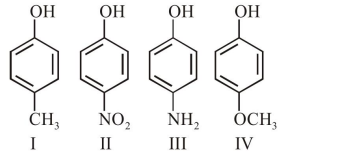1. $\mathrm{I}<\mathrm{IV}<\mathrm{III}<\mathrm{II}$

2. IV $<$ I $<$ II $<$ III

3. $\mathrm{I}<\mathrm{III}<\mathrm{IV}<\mathrm{II}$

4. $\mathrm{III}<\mathrm{I}<\mathrm{II}<\mathrm{IV}$

Correct Option: 1,

Solution: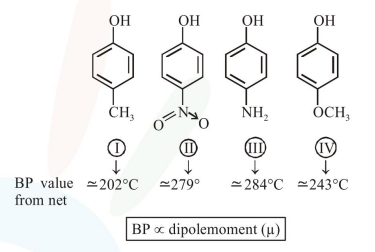Increasing order of boiling point is :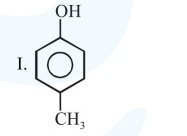$\Rightarrow$ Shows hydrogen bonding from $-\mathrm{O}-\mathrm{H}$ group only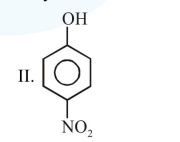$\Rightarrow$ Shows strongest hydrogen bonding from both sides of $-\mathrm{OH}$ group as well as $-\mathrm{NO}_{2}$ group.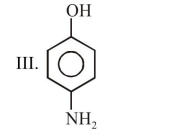$\Rightarrow$ Shows stronger hydrogen from both side of $-\mathrm{OH}$ group as well as $-\mathrm{NH}_{2}$ group.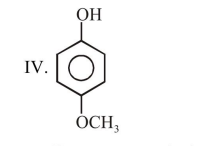$\Rightarrow$ Shows stronger hydrogen bonding from one side $-\mathrm{OH}-$ group and another side of $-\mathrm{OCH}_{3}$ group shows only dipole-dipole interaction.

$\Rightarrow$ Hence correct order of boiling point is:

(I) $<$ (IV) $<$ (III) $<$ (II)